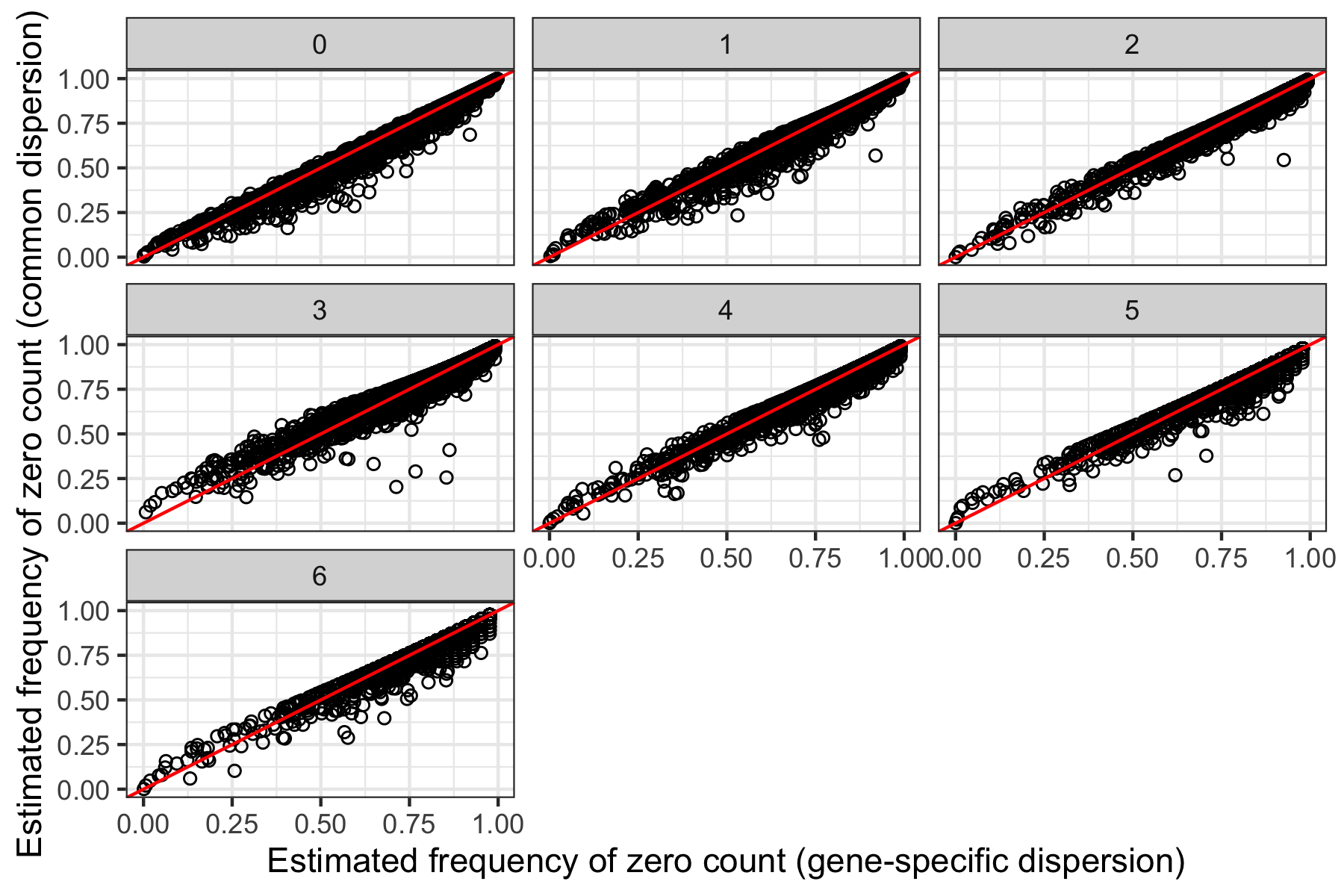# Introduction to PhitestR

#### 2021-07-27

PhitestR currently supports two types of input: a UMI count matrix with cluster labels, or a Seurat object with cluster information.

### Run PhitestR with a count matrix

To demonstrate the usage of PhitestR with a count matrix, we use an example Cortex dataset which can be downloaded from this link. To use PhitestR, we need a count matrix (in this example, object) with rows representing genes and columns representing cells. In addition, we also need a (character or numeric) vector of labels (in this case, label) specifying the assignments of the cells.

data = readRDS("Cortex2_count.rds")
object = data$count label = data$labels

Now, to run Phitest, we just need to specify the count matrix, cluster labels, and the number of cores to be used in parallel computation. The result is a list containing two elements. The first element is a vector of values corresponding to the clusters in label.

result = phitest(object, label, ncores = 2)
result$pval #Oligodendrocyte Endothelial Astrocyte # 0.6107304 0.9999999 0.4844792  The second element is a named list of estimated parameters corresponding to the clusters. The list names are the cluster labels, and each element is a matrix with rows representing genes and columns representing estimated parameters. ### Run PhitestR with a Seurat object To demonstrate the usage of PhitestR with a Seurat object, we use an example Cortex dataset which can be downloaded from this link. This Cortex count matrix has been analyzed following Seurat’s tutorial. Suppose we use Seurat to cluster the cells with a resolution of 0.1, we can check if the clustering is successful by checking the seurat_clusters entry in the object. This entry is required in order to use Phitest. data = readRDS("Cortex2_Seurat.rds") object = FindClusters(data, resolution = 0.1) table(object$seurat_clusters)

Now, to run Phitest, we just need to specify the Seurat object and the number of cores to be used in parallel computation. The result is a list containing two elements. The first element is a vector of values corresponding to the clusters in object$seurat_clusters. For example, the first three values correspond to cluster 4, 1, and 0, respectively. result2 = phitest(object, ncores = 2) result2$pval[1:3]
#           4            1            0
#1.000000e+00 9.997925e-01 1.549529e-08

The second element is a named list of estimated parameters corresponding to the clusters. The list names are the cluster labels, and each element is a matrix with rows representing genes and columns representing estimated parameters. For example, we could make similar plots as shown in the paper with the code below:

library(ggplot2)
labels = names(result2$par) par = lapply(labels, function(l){ tp = result2$par[[l]]
tp\$cluster = l
return(tp)
})
par = Reduce(rbind, par)
ggplot(par, aes(x = dhat, y = dhat.c)) +
geom_point(shape = 1) +
geom_abline(intercept = 0, slope = 1, color = "red") +
facet_wrap(~cluster) +
xlab("Estimated frequency of zero count (gene-specific dispersion)") +
ylab("Estimated frequency of zero count (common dispersion)")Estimated parameters of Phitest by clusters.